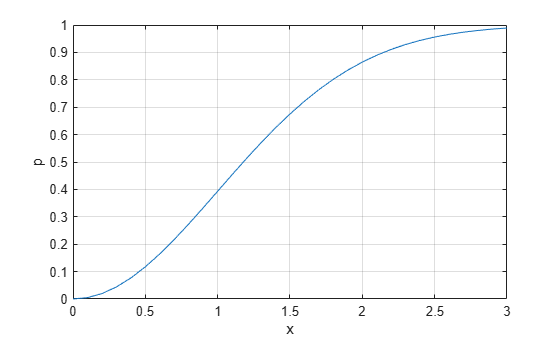Documentation

# raylcdf

Rayleigh cumulative distribution function

## Syntax

```p = raylcdf(x,b) p = raylcdf(x,b,'upper') ```

## Description

`p = raylcdf(x,b)` returns the Rayleigh cdf at each value in `x` using the corresponding scale parameter, `b`. `x` and `b` can be vectors, matrices, or multidimensional arrays that all have the same size. A scalar input for `x` or `b` is expanded to a constant array with the same dimensions as the other input.

`p = raylcdf(x,b,'upper')` returns the complement of the Rayleigh cdf at each value in `x`, using an algorithm that more accurately computes the extreme upper tail probabilities.

The Rayleigh cdf is

`$y=F\left(x|b\right)={\int }_{0}^{x}\frac{t}{{b}^{2}}{e}^{\left(\frac{-{t}^{2}}{2{b}^{2}}\right)}dt$`

## Examples

collapse all

Compute the cdf of a Rayleigh distribution with parameter `B = 1`.

```x = 0:0.1:3; p = raylcdf(x,1);```

Plot the cdf.

```figure; plot(x,p)```## References

 Evans, M., N. Hastings, and B. Peacock. Statistical Distributions. Hoboken, NJ: Wiley-Interscience, 2000. pp. 134–136.